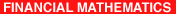ERCIM News No.38 - July 1999

## Stochastic Methods in Finance: Evaluating Predictions

by Valeria Skrivankova

Stochastic methods in finance are mainly connected with risky financial operations, for example the security market trading. Relevant decisions are affected by a prediction of some quantity, but the adequate judgment on the future fulfilments of the expectation is often a difficult problem. Common methods of the evaluation of judgments are based on long term observations. The presented method of evaluation called Reflexive Evaluation Of Predictive Expertises (REOPE) is also applicable for the unrepeatable expertises.

The financial market models are based on the premise that investors like return and dislike risk. So the financial management wants to maximize the return and minimize the risk. For this purpose it is necessary to have the best forecast of expected return and risk. The definition of risk used in a classical Markowitz ‘Mean-Variance’ Model for effective portfolio is a measure of the variability of return called the standard deviation of return. So the main task is to predict (estimate) the expected return and the standard deviation.

What Forecasting can and cannot do?

One should not expect any forecasting theory or technique to predict the precise value at which a future price will settle tomorrow, or any given day, or what the exact high or low will be. A good forecasting method will on average have a small forecast error; that is, the difference between the forecast price and the actual market price will be small. Further, the forecast must be unbiased, which means that the errors should overshoot the actual price as often and by as much as they undershoot it.
Measuring Talent by Common Methods

Talent can be differentiated from luck only by examining results averaged over many periods. Investors and management cannot afford to evaluate future performance and the reason for it merely on the basis of a one period forecast. They must consider such things as the expected contribution of a security analyst over time, how to estimate it and how to organize to make the most of it.

Formulation of the Problem for REOPE

Consider the predicted quantity as a random variable X (eg portfolio return). Suppose that the quality of the judgement of X is evaluated according to the correspondence of the estimation with the consequently realized value of X only. Let t(X) be a relevant parameter of the distribution of X in sense of expert’s opinion. The problem of the judgement evaluation is generally based on an evaluation function
h = h(x,estim t), where x is the realized value of X and estim t is the expert’s estimation of t. The expert’s criterion of optimality is fulfilled if he gives unbiased estimate of t. Suppose that estim t is fully determined by the expert’s effort to optimize his criterion C which is connected with the evaluation
h(X,estim t) of his work only. So we have to find the concrete evaluation function as a solution of certain equation. The expert’s perfomance evaluation:

• optimizes the expert’s criterion of utility C if he delivers an unbiased judgement
• reflects the correspondence between the single estimation of some parameter and the consequently realized value of the predicted quantity only
• motivates the expert to put a reasonable deal of his effort in the judgement.

Mean Value Judgements

Let X be the followed random variable, E represents the mean value operator, the parameter t of the distribution of X is E(X). The expert’s criterion of optimality consists in the maximization of the mean value of his future evaluation here. We search for a function h so that E[h(X,E(X))] is the maximum of E[h(X,estim t)]. We can show that the function h given as a - b(estim t - x).(estim t - x), where a,x are real numbers and b positive, fulfils our condition. Parameters a,b can be choosen by higher level management (management of expertises).

Common methods for the evaluation of judgements are based on statistical analysis of adequacy of past judgement. Ferguson (1975) uses simple regression methods which require long term observations. These models aren’t suitable for the unrepeatable expertises. The presented method of evaluation is always applicable if the manager knows the expert’s criterion C and the expert knows the evaluation function h . This method reflects the expert’s success immediately so motivates him to the optimal performance in every judgement.

The given solution of the problem does not claim completeness. Probability distribution judgements and manager’s utility optimization were published by Skrivanek (1996) and Skrivankova (1998). Statistical regulation of estimations and hypothesis testing of their convenience are studied.